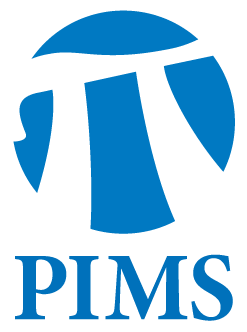## Discrete Math Seminar: Cesar Hernandez-Cruz

• Date: 04/24/2012
• Time: 14:30
Lecturer(s):
Cesar Hernandez-Cruz
Location:

Simon Fraser University

Topic:

(k,l)-kernels of digraphs

Description:

Abstract:

Let D=(V,A) be adigraph. A subset K of V is k-indepenent if the (directed) distance betweenevery pair of vertices in K is at least k, we say that K is l-absorbent ifevery vertex not in K reaches a vertex in K at distance at most l and we call K a (k,l)-kernel of D if it is k-independent and l-absorbent. A k-kernelis a (k,k-1)-kernel, and a kernel is a 2-kernel.Kernels of digraphs have been widely studied since their introduction by von Neumann and Morgenstern in 1944 and different generalizations have been proposed and studied, but (k,l)-kernels have received small attention so far. This talk is intendedto be a brief introduction to the subject, highlighting some existing results and open problems, finalizing with very recent results about 3-kernels, e.g., a proof of the NP-completness of the problem of deciding whether ornot a digraph with circumference 6 and homomorphic to a directed triangle has a 3-kernel.

Other Information: